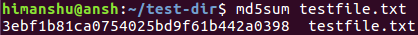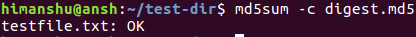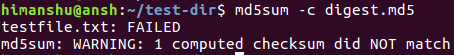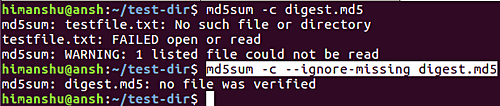Linux md5sum Command Tutorial for Beginners (5 Examples)

While we have already discussed the cksum command line utility, there's another tool that you can use in scenarios where, say, you need to verify the integrity of files during transfers. The tool we're talking about here is md5sum. In this tutorial, we will discuss the basics of this command using some easy to understand examples.

Bue before we do that, it's worth mentioning that all examples in this article have been tested on Ubuntu 16.04 LTS.

Linux md5sum command

The md5sum command basically computes and checks MD5 (128-bit) message digest for files. Here's the syntax of the command:

md5sum [OPTION]... [FILE]...

The following Q&A-styled examples should give you a better idea of how md5sum works.

Q1. How md5sum command works?

Basic usage is pretty simple - if you want to compute MD5 checksum for a file, all you have to do is to pass the name of the file as input to the command. For example:

md5sum testfile.txtYou can also redirect the output to a text file so that you can later check the digest against any change/corruption in the file.

md5sum test.txt > digest.md5

Use the -c command line option to check the digest.

md5sum -c digest.md5And if there's any change or corruption, here's the kind of output md5sum produces:Q2. How to change way files are read checksum is created?

By default, the md5sum command reads input in text mode. However, if you want, you can make the tool read input in binary mode as well. This you can do using the -b command line option.

md5sum -b [filename]

Plus, you can also force md5sum to create a BSD-style checksum using the --tag command line option.

Q3. How to make md5sum ignore missing files?

While verifying checksums, if you want md5sum to neither fail nor report status for missing files, then you can use the --ignore-missing option. Following screenshot shows this option in action:So you can see that the error and the notification wasn't produced in the second case.

Q4. How to make md5sum avoid printing OK for each successfully verified file?

Following is the default behavior of md5sum:However, if you do not want to see OK for each successfully verified file, you can use the --quiet option. So in our case, the above command would become:

md5sum -c --quiet digest.md5

Q5. How md5sum computes checksums?

The sums are computed by the tool as described in RFC 1321. Here's what the man page says:

The  sums  are  computed  as described in RFC 1321.  When checking, the
input should be a former output of this program.  The default  mode  is
to  print  a  line with checksum, a space, a character indicating input
mode ('*' for binary, ' ' for text or where binary  is  insignificant),
and name for each FILE.

The MD5 algorithm should not be used any more for security related pur?
poses.  Instead, better use an SHA-2 algorithm, implemented in the pro?
grams sha224sum(1), sha256sum(1), sha384sum(1), sha512sum(1)

Conclusion

If you are a Linux command line newbie, there are fewer chances that you'll use md5sum in your early days. It's primarily aimed at system admins/pro users. But there's no harm in developing a basic understanding of how the tool works, which is precisely what this tutorial is focused at. In case you want to know more, you can head to md5sum's man page.view as pdf |print